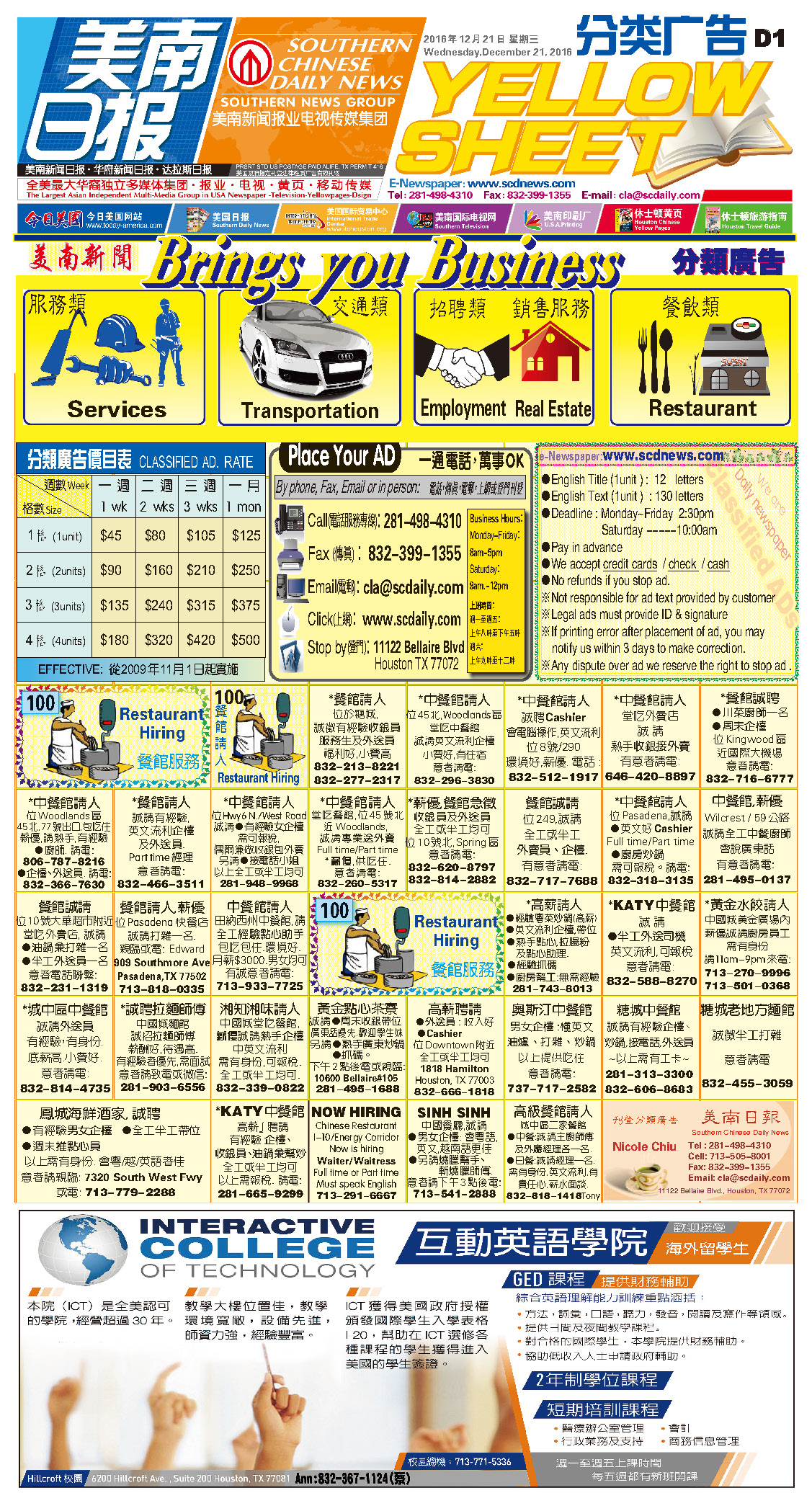161231 Epaper

 A Section B SectionC Section D Section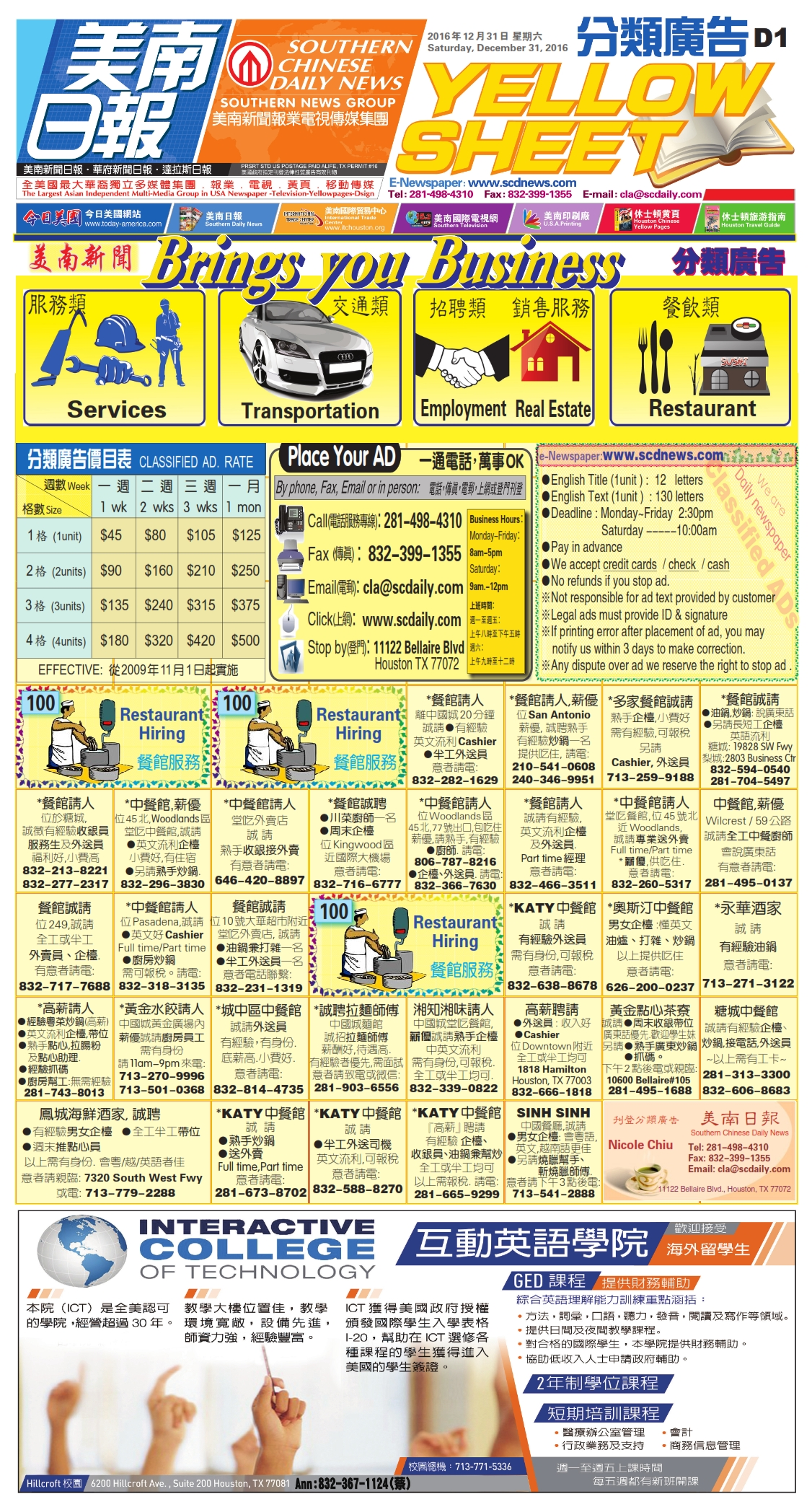161230 Epaper

 A Section B SectionC Section D Section161229 Epaper

 A Section &1612nbsp; B SectionC Section D Section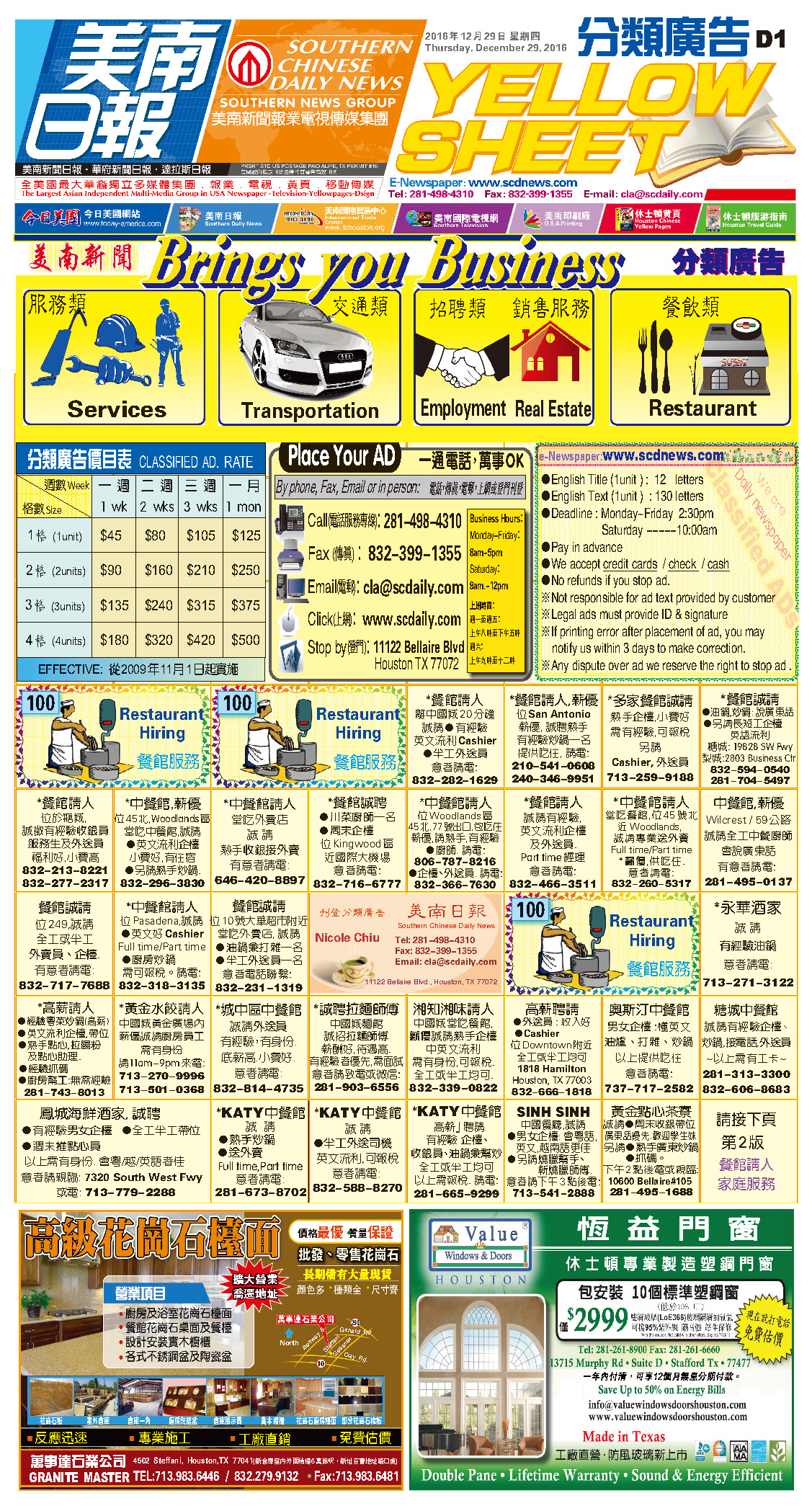161228 Epaper

 A Section &1612nbsp; B SectionC Section D Section161227 Epaper

 A Section &1612nbsp; B SectionC Section D Section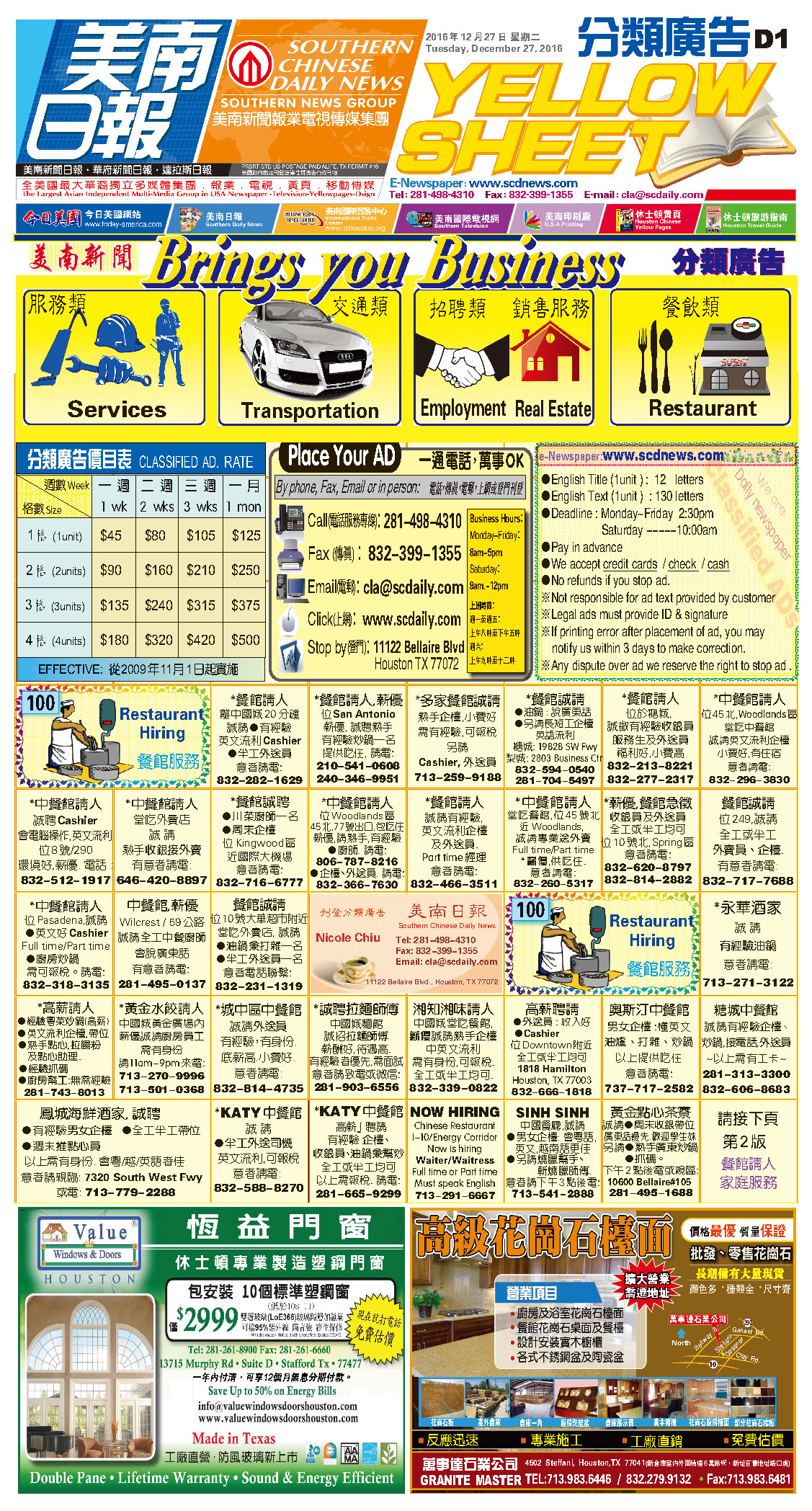161226 Epaper

 A Section &1612nbsp; B SectionC Section D Section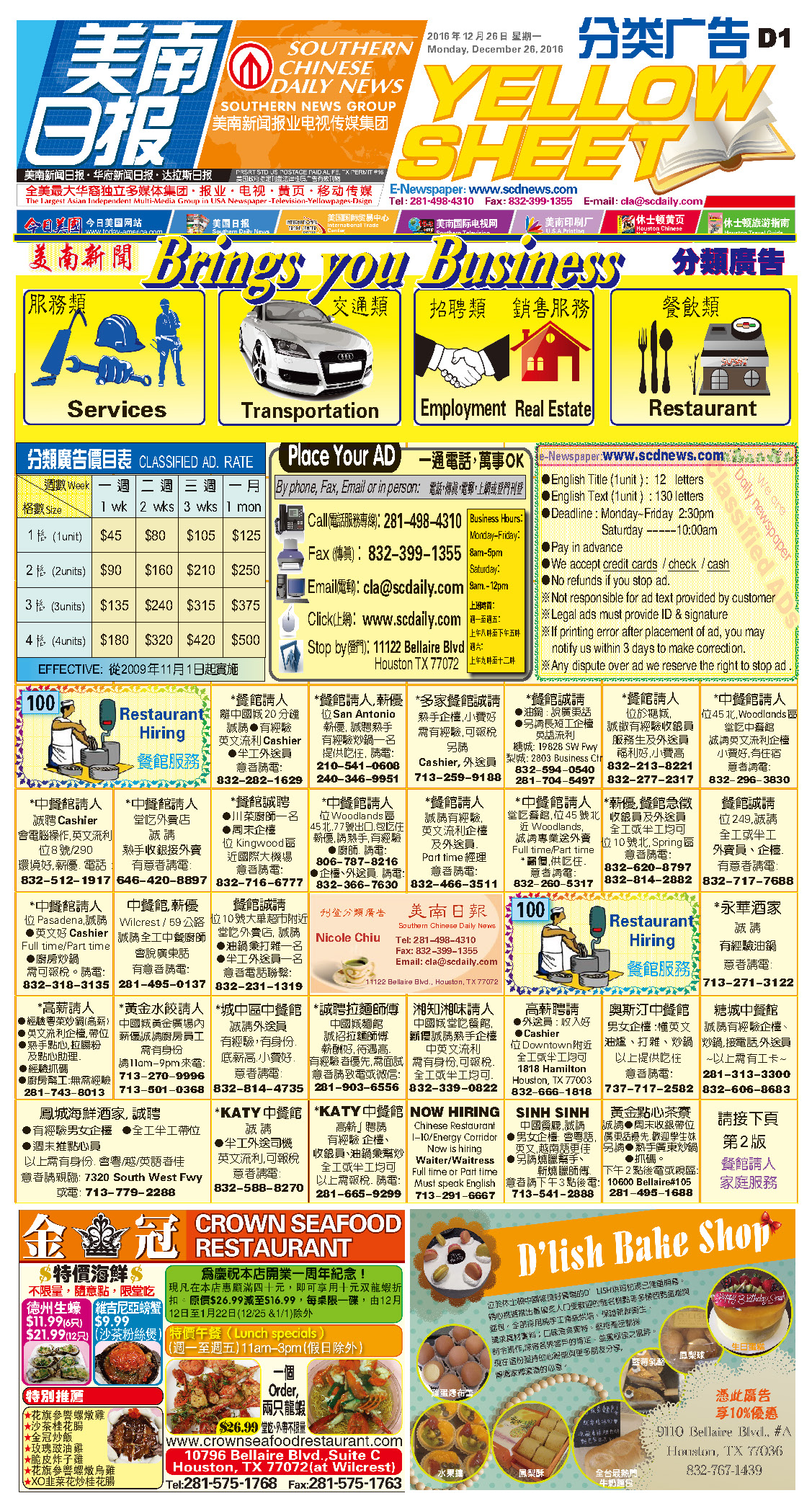161225 Epaper

 A Section &1612nbsp; B SectionC Section D Section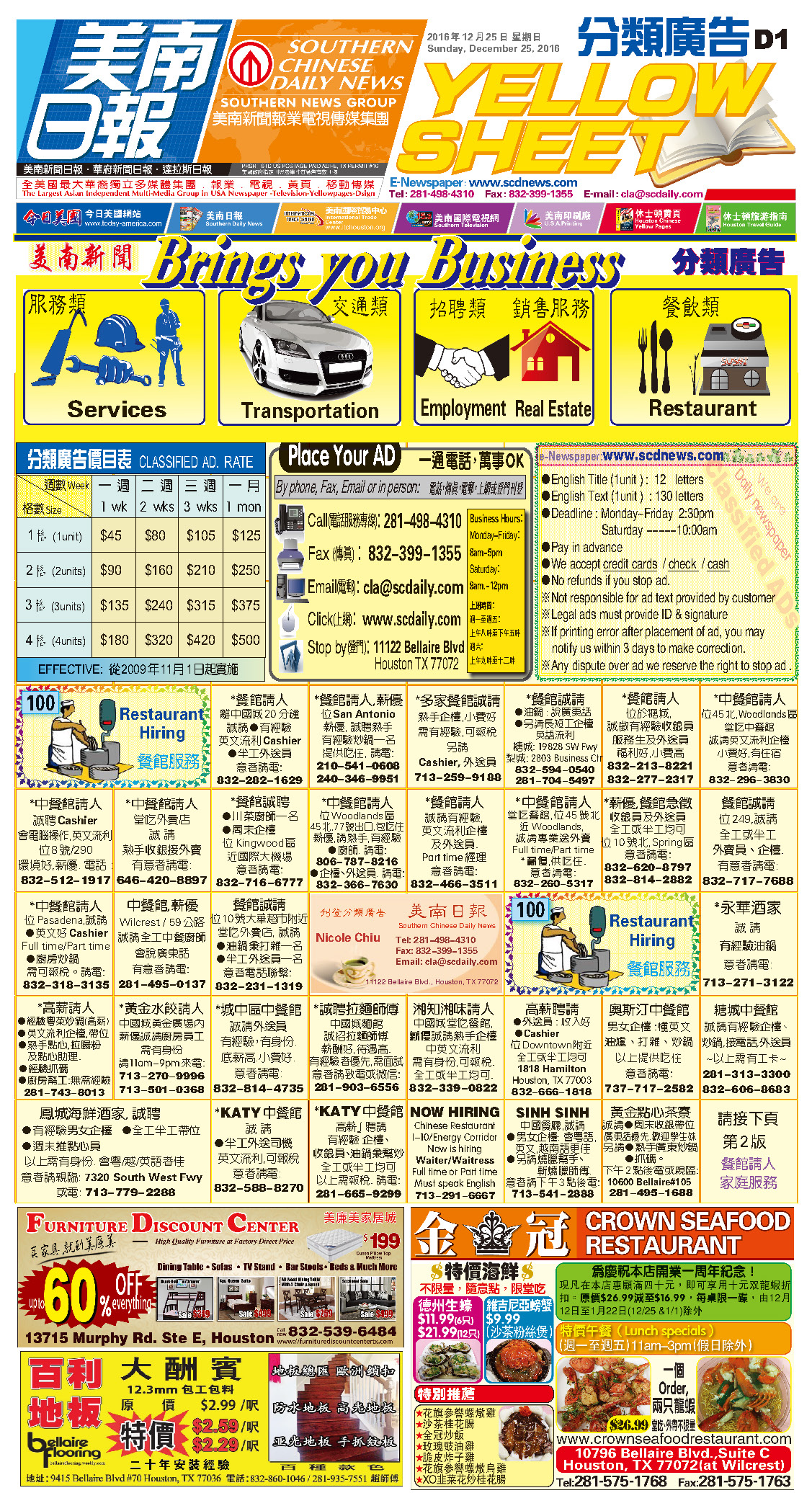161224 Epaper

 A Section &1612nbsp; B SectionC Section D Section161222 Epaper

 A Section B SectionC Section D Section161223 Epaper

 A Section B SectionC Section D Section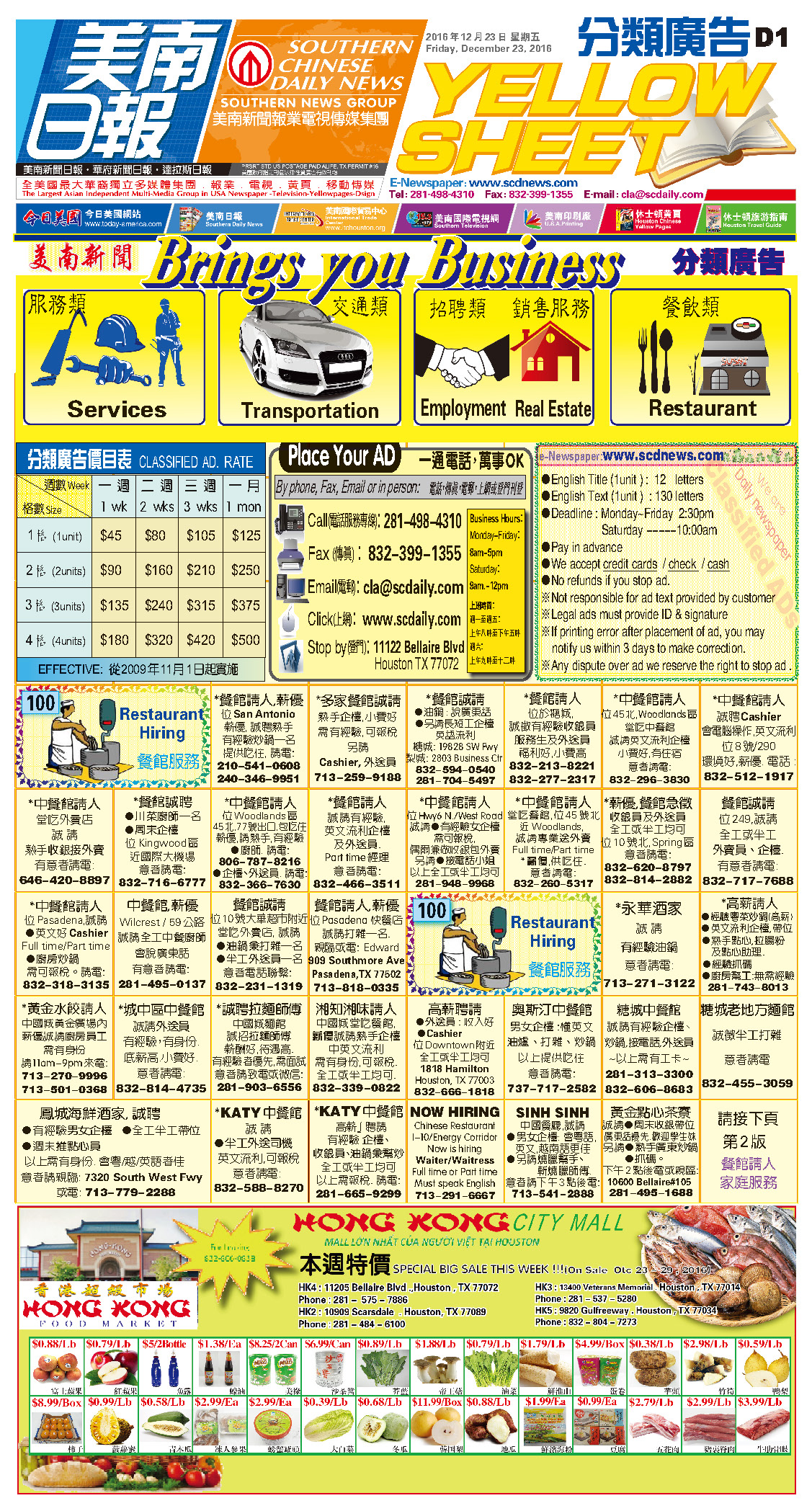161221 Epaper

 A Section B SectionC Section D Section